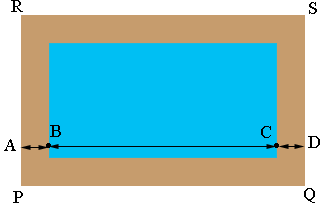SEARCH HOMEMath Central Quandaries & QueriesQuestion from Anita, a parent: The measurement of a swimming pool are 32 feet by 18 feet. How much fencing is needed if the fence is to be built 7 feet from each side of the pool?Anita,The distance from B to C is 32 feet. The distance from A to B is 7 feet. The distance from C to D is 7 feet. What is the length of PQ? What is the length of RS? That is the two long sides of fence that you need. What is the length of the two short sides?

PennyMath Central is supported by the University of Regina and The Pacific Institute for the Mathematical Sciences.